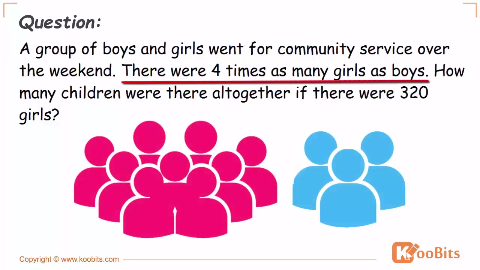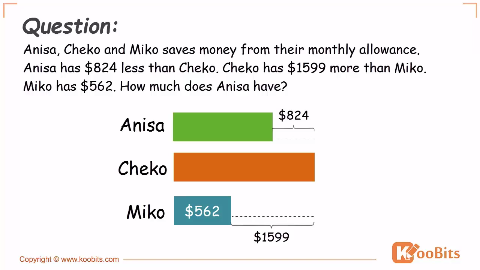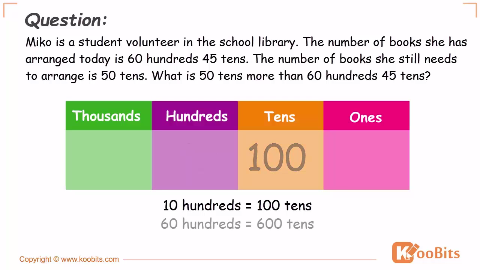# Secrets to Solving the 11 Types of Problem Sums

Have you ever wondered why your child’s problem sums are so complicated? Problem sums, or math word problems, are designed not to test basic mathematical ability but to impart the knowledge of various concepts. In fact, there are a total of 11 different types of problem sums, and these can be found across all the different math topics.

## For Parents: Best Way To Teach Problem Sums At Home– 100,000+ MOE Syllabus Aligned Maths Questions with Solutions– 500+ Professional Singapore Maths Bar Model Method Videos## 1. Remainder Concept

Remainder concept problem sums often contain the word “remainder” or ask about the total amount of something given the remainder. Most of the time, remainder concept questions test the knowledge of fractions.

###### Example Question

Mrs Lim had some chocolates. She gave 178 chocolates to her neighbour and 2/5 of the remainder to her daughter. With the remaining chocolates, she gave 1/3 of the chocolates to her son and then had 256 chocolates left. How many chocolates had she at first?

## 2. Repeated Identity Concept

Repeated Identity concept problem sums commonly feature one unknown or variable as a point of reference for other unknowns. Hence, the units method is used to solve these problem sums.

###### Example Question

Alice, Betty, Clara and Denise shared \$168. Denise received 1/7 of the total amount of money received by Alice, Clara and Betty. Alice received 3/4 of the total amount of money received by Clara and Betty. Betty received 2/5 as much as Clara. How much did Betty receive?

## 3. Equal Concept

Equal Concept problem sums compare fractions or percentages from different unknowns, but which represent equal amounts.

## 4. External Transfer (unchanged quantity)

There are four different transfer type problem sums, and it can be difficult for kids to determine which transfer type is being used in the question. The simplest way to solve transfer type problem sums is to draw models. However, children who are more advanced can try using algebra and simultaneous equations to derive the answer.

In an external transfer with unchanged quantity, one variable has amounts added to or subtracted from it while the other remains unchanged.

###### Example Question

Mrs Lim baked thrice as many cakes as Mrs Loh. After Mrs Lim gave away 115cakes, Mrs Loh had twice as many cakes as her. How many cakes did Mrs Lim have left?

## 5. Internal Transfer

Internal transfer concept problem sums refers to questions where an amount is subtracted from one unknown and added to another, so the total amount in question remains unchanged. It is also known as the Constant Total concept.

###### Example Question

Leon and his sister Lily have a total of 120 crayons. If Leon gives Lily 5 crayons, Lily will have nine times as many crayons as Leon. How many more crayons does Lily have than Leon?

## 6. External Transfer (same difference)

In this type of external transfer, the same amount is being transferred to or from the variables/unknowns. Hence, the difference between the unknowns remains unchanged. Such questions are almost always used to test knowledge of ratios.

###### Example Question

Question: Ryan is 33 years old and his son is 5 years old now. In how many years will Ryan be thrice as old as his son?

In such problem sums, remember that age difference between two people will always remain a constant. Also, when drawing models to solve the question, It is easier to draw the final model – where the ratio is known – and then work backwards.

## 7. External Transfer (changed quantity)

In an external transfer with changed quantity,  both variables or unknowns have amounts added to or subtracted from them. Hence, it is difficult to solve such problem sums using the model method.

###### Example Question

The number of ten-cent coins in a box was 1/2 the number of fifty-cent coins. Syed took out 5 fifty-cent coins and exchanged them for ten-cent coins. Then he put the money back into the box. The number of fifty-cent coins became 5/8 the number of ten-cent coins. How much money was there in the box?

The best method to use for solving external transfer (changed quantity) questions is the units method. This question is particularly tricky because it involves the counting of money as well as the number of coins.

## 8. Pattern Concept

Pattern concept problem sums are among the most challenging, as they require students to recognize varying number patterns that may involve more than one arithmetic operation.

###### Example Question

Study the pattern below:
Pattern 1                Pattern 2               Pattern 3(a) Complete the table below.

Pattern Number
1
2
3
4
Matchsticks
4
7
10
___

(b) How many matchsticks are there in Pattern 11?
(c) Which pattern will have 76 matchsticks?

Here, you will need to find the relation between the pattern number and the number of matchsticks used (Pattern Number * 3 + 1). Order of operations is also important for getting the right answer.

## 9. Part-whole Concept (Proportions)

The part-whole concept is one of the main concepts for problem sums, and forms the basis for other concepts mentioned here. Read more about it in our detailed post about the Singapore math model method.

## 10. Simultaneous Concept

Simultaneous concept problem sums are a precursor to simultaneous equations in algebra, where abstract variables are represented using objects. While simultaneous concept questions can be solved using the model method, they are the best for introducing your child to algebraic methods.

###### Example Question

3 files and 2 books cost \$60. 2 files and 3 books cost \$70. Find the cost of 1 file.

## 11. Gap Concept / Difference Concept

Gap concept problem sums are based on the difference between one variable and another. They can be solved using the ever-useful model method, or by arithmetic.

###### Example Question

Joe’s father had given Joe and Kevin an equal amount of money. Joe spent \$20 each day and Kevin spent \$25 each day. When Joe had \$157 left, Kevin had \$82 left. How much did Kevin receive?

• Each day, Kevin spends \$25 – \$20 = \$5 more than Joe.
• After some days, the difference in money left is \$157 – \$82 = \$75.
• So, this money was spent over 75 ÷ 5 = 15 days.
• Kevin received \$82 left + 25 × 15 days = \$457.

You can also encourage your child to double-check his or her answer by making sure Joe also receives the same amount of money.

Remember you should try also rewarding activities after practice.

•Gohgoal

How to solve this problem sums :
Geeta gets 50 cents more pocket money than Hani every day. Each of them spends 60 cents a day and saves the rest. If Hani saves \$40, Geeta will have saved \$20 more than Hani. How much is hani’s daily pocket money?

•CarolLin

Since the amount they spend each day is the same, the difference in the amount saved each day is still 50 cents.
If Geeta saved \$20 more than Hani,
No. of days they saved for = \$20 / \$0.50 = 40
Amt. saved by Hani each day = \$40 / 40 = \$1
Hani’s daily pocket money = \$1 + \$0.60 = \$1.60

•Ace

Library A has 50 more books than library b. Library b will have twice amount of books than library A when 120 books are transferred to library b from library A. How many books are there altogether?

•FyMath

@Ace I think this question has a problem.

A has more than B at first.

If books were transferred from B  to A, then B should have a lot lesser.

How can B have two times as much as A in the end?

•abc123

FyMath hi the question is saying to B from A. This question tricked you with English.

•JayaSanthi

Johan
had some \$5 notes and \$2 notes.The
ratio of the number of \$5 notes to the number of \$2 notes was 6 : 11.When he exchanged 10 pieces of \$5 notes for
some \$2 notes, the ratio of the number of \$5 notes to the number of \$2 notes
became 2 : 7.How much money did Johan
have altogether?

•Sarker

@Ace  120 – 50 = 70 = half of B

B has 140
A has 140 + 50 = 190
Total 190 +140 = 330

•Sarker

10 \$5 notes = 25 \$2 notes
6:11
Now 6U – 10 : 11U +25= 2 : 7
U = 6
Was 36 X .5 = 18; 66 X .2 = 13.2, Total = 31.2
Now 36-10 = 26; 26X .5 = 13; 66+25 = 91; 91X.2 == 18.2; total 31.2

•Sarker

JayaSanthi  http://www.koobits.com/ 5ptsFeatured
3 minutes ago
10 \$5 notes = 25 \$2 notes
6:11
Now 6U – 10 : 11U +25= 2 : 7
U = 6
Was 36 X .5 = 18; 66 X .2 = 13.2, Total = 31.2
Now 36-10 = 26; 26X .5 = 13; 66+25 = 91; 91X.2 == 18.2; total 31.2

•Sarker

@Ace  The earlier answer was wrong
The correct way follows:
Ace  120 – 50 = 70
Now after the transfer the half of B = 70 + 120 = 190
So B has 380
And A has 190

And B had 260; Total 570

•kocoakitten

On Monday, there were 280 more chairs in hall B than hall A . On Tuesday, 0.25 of the chairs were moved from hall B to hall A. On Wednesday, 0.2 of the chairs were moved back to hall B. On Thursday, half of the chairs in hall B were moved back again to hall A. In the end, there were 520 more chairs in hall A than hall B. How many chairs were there in hall B at first???

•Infernocreep

This isn’t too hard. All you have to do is draw the model for all of the days. 0.25 is 1/4 so i draw 4 units for the model of hall B in Monday, then i draw a second bar  in the same model which represents 280. Then I draw the model for hall A, which is just 4 units. On Thursday, there will be a difference of 520.Hall B will have 2 units+ 112, while hall A will have 6 units+ 170. After that you will have to find 4 units. First, subtract 112 from 520, then add the answer with 280,and BOOM you have your answer.

•AGNESLIEW

In a school, 40% of the pupils and 10 pupils go to school by car. 25% of the remaining and another 8 pupils
walk to school and the rest of them go to school by bus. If there are 152 pupils walk to school, how many pupils
go to school by bus ?

•HogRiderR6

AGNESLIEW  This is really a trick question. You do not need to solve how many go by car.
152-8=144(25%)
75% is thus 144*3= 432
Bus is thus 432-8=424 (ans)
P.S. I am a 10 year old not kidding

•girllol

@HogRiderR6 Dun boast lah. 10 yr old so wat?

•girllol

Example 11. also can use algebra 2 solve.

•VenusTan

A group of girls shared some stickers among themselves.They tried taking 17 stickers each,but found that the last girl had only 3 stickers.
When each girl took 15 stickers,there were 6 stickers left over.
a)How many girls were there?
b)How many stickers were there althogether?